• 在做leetcode题目（https://oj.leetcode.com/problemset/algorithms/）的时候，我们总要自己写测试用例，常常要生成vector, list, tree，我将这些常用操作封装了一下，方便大家使用

在做leetcode题目（https://oj.leetcode.com/problemset/algorithms/）的时候，我们总要自己写测试用例，常常要生成vector, list, tree，我将这些常用操作封装了一下，方便大家使用
tool.h
//tool.h
#ifndef TOOL_H_
#define TOOL_H_

#include <iostream>
#include <vector>
#include <cstdarg>
#include <string>
using namespace std;

#include "windows.h"

struct ListNode
{
int val;
ListNode *next;
ListNode(int x) : val(x), next(NULL) {}
};

struct TreeNode {
int val;
TreeNode *left;
TreeNode *right;
TreeNode(int x=0) : val(x), left(NULL), right(NULL) {}
};

void ArrayPrint(int arr[], int count);

vector<int> IntVectorCreate(int count, ...);
void IntVectorPrint(const vector<int> &vec);
void IntVector2DPrint(const vector<vector<int>> &vec);

vector<string> StringVectorCreate(int count, ...);
void StringVectorPrint(const vector<string> &vec);

TreeNode *TreeCreate(int count, ...);
void TreePrint(TreeNode *root);

ListNode *ListCreate(int count, ...);

#endiftool.cpp//tool.cpp
#include "tool.h"

{
while (node)
{
cout << node->val << " -> ";
node = node->next;
}
cout << endl;
}

ListNode *ListCreate(int count, ...)
{
va_list argptr;
va_start(argptr, count);

int num = va_arg(argptr, int);
for (int i = 1; i < count; i++)
{
num = va_arg(argptr, int);
ListNode *temp = new ListNode(num);
curr->next = temp;
curr = temp;
}
va_end(argptr);
}

void ArrayPrint(int arr[], int count)
{
for (int i = 0; i < count; i++)
cout << arr[i] << " , ";
cout << endl;
}

void IntVectorPrint(const vector<int> &vec)
{
for (size_t i = 0; i < vec.size(); i++)
cout << vec[i] << " , ";
cout << endl;
}

void IntVector2DPrint(const vector<vector<int>> &vec)
{
printf("[\n");
for (size_t i = 0; i < vec.size(); i++)
{
printf("[ ");
for (size_t j = 0; j < vec[i].size(); j++)
printf("%d, ", vec[i][j]);
printf(" ]\n");
}
printf("]\n");
}

void StringVectorPrint(const vector<string> &vec)
{
cout << "------------------------------------" << endl;
for (size_t i = 0; i < vec.size(); i++)
cout << vec[i] << "_" << endl;
cout << "------------------------------------" << endl;
}

//

vector<int> IntVectorCreate(int count, ...)
{
vector<int> result;
va_list argptr;
va_start(argptr, count);

for(int i=0; i<count; i++)
{
int num = va_arg(argptr, int);
result.push_back(num);
}
va_end(argptr);
return result;
}

vector<string> StringVectorCreate(int count, ...)
{
vector<string> result;
va_list argptr;
va_start(argptr, count);

for (int i = 0; i < count; i++)
{
char *str = va_arg(argptr, char*);
result.push_back(str);
}
va_end(argptr);
return result;
}

//

TreeNode *TreeCreate(int count, ...)
{
va_list argptr;
va_start(argptr, count);

TreeNode **treeArr = new TreeNode*[count];
for (int i = 0; i < count; i++)
{
int num = va_arg(argptr, int);
if (INT_MAX == num)
treeArr[i] = NULL;
else
treeArr[i] = new TreeNode(num);
}

int curr = 1;
for (int i = 0; i<count; i++)
{
if( !treeArr[i] )
continue;

if( curr < count )
treeArr[i]->left = treeArr[curr++];
if (curr < count)
treeArr[i]->right = treeArr[curr++];
}

va_end(argptr);
return treeArr;
}

void SubTreePrint(TreeNode *node, int level)
{
if ( !node )
return;

SubTreePrint(node->right, level + 1);
for (int i = 0; i < level; i++)
printf("    ");
printf("%04d\n", node->val);
SubTreePrint(node->left, level + 1);
}

void TreePrint(TreeNode *root)
{
cout << "------------------------------------" << endl;
SubTreePrint(root, 0);
cout << "------------------------------------" << endl;
}
array, vector, list这几个结构的Create和Print很简单，就不多解释了
PS: XXXCreate函数的第一个参数总是元素的个数
int main()
{
int arr[] = { 1, 2, 3, 4, 5 };
ArrayPrint(arr, 5);

vector<int> ivec = IntVectorCreate(5, 1, 2, 3, 4, 5);
IntVectorPrint(ivec);

vector<string> svec = StringVectorCreate(5, "11", "22", "33", "44", "55");
StringVectorPrint(svec);

ListNode *node = ListCreate(5, 1, 2, 3, 4, 5);
ListPrint(node);

getchar();
return 0;
}

tree的情况比较复杂，详细解释一下
假设一棵树如下：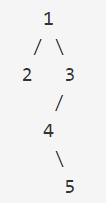那么leetcode上的表示是：{1,2,3,#,#,4,#,#,5}
代码是这个样子的：（#用INT_MAX代替）
    TreeNode *tree = TreeCreate(9, 1, 2, 3, INT_MAX, INT_MAX, 4, INT_MAX, INT_MAX, 5);
TreePrint(tree);

打印出来之后，就是这个样子的一切搞定

展开全文• 实写构造函数leetcode leetcode在线练习，这是一个算法设计套路，可以帮助开发者挖掘潜力，提高生产力，将推动开发者每天投入到应对测验中。 如何加入 找到合适的位置 您的工作区可能位于leetcode/src/username/ ...
• 力扣的测试题 数组 1.删除排序数组中的重复项 给定一个排序数组，你需要在 原地 删除重复出现的元素，使得每个元素只出现一次，返回移除后数组的新长度。 不要使用额外的数组空间，你必须在 原地 修改输入数组 并在...
• 前言：代码写的挺随意的，当初写的时候都是赶着赶紧测试。。。只要实现了这个功能就好。不保证边际用例和最高效率 但使用应该是拿来就能用。就我测试过的题而言，这些够用了 二维char工厂 public class CharArr_Two...
文章目录LeetCode自定义的数据结构生成方法
完整代码github地址

LeetCode自定义的数据结构


public class UtilDS {
public static class ListNode {
public int val;
public ListNode next;

public ListNode(int x) {
val = x;
}
}

public static class TreeNode {
public int val;
public TreeNode left;
public TreeNode right;

public TreeNode(int x) {
val = x;
}
}

}


生成方法
首先是工厂
public interface TestHelperFactory<T> {
T produce(String s);
}


前言：代码写的挺随意的，当初写的时候都是赶着赶紧测试。。。只要实现了这个功能就好。不保证边际用例和最高效率
但使用应该是拿来就能用。就我测试过的题而言，这些够用了

二维char工厂
public class CharArr_Two_Factory implements TestHelperFactory<char[][]> {
@Override
public char[][] produce(String s) {
s = s.replace("\"", "");
int n = s.length();
Stack<Character> s1 = new Stack<Character>();
Stack<Character> s2 = new Stack<Character>();
int m = n;
for (int i = 0; i < n; ++i) {
char c = s.charAt(i);
if (c == ',')
continue;
if (c != ']') {
} else {
while (!s1.isEmpty()) {
char c1 = s1.pop();
if (c1 != '[') {
}
}
int size = s2.size();
if (size == 0) {
continue;
}
m = size;
char[] cArr = new char[size];
for (int j = 0; j < m; ++j) {
cArr[j] = s2.pop();
}
}
}
int qSize = queue.size();
char[][] res = new char[qSize][m];
for (int i = 0; i < qSize; ++i) {
char[] temp = queue.poll();
res[i] = temp;
}
return res;
}

一维数组工厂
public class IntArr_One_Factory implements TestHelperFactory<int[]> {

@Override
public int[] produce(String s) {
String temp1 = s.replace("[", "");
String temp2 = temp1.replace("]", "");
if(temp2.length()==0){
return new int;
}
String[] s2 = temp2.split(",");
int n = s2.length;
int[] res = new int[n];
for (int i = 0; i < n; ++i) {
res[i] = Integer.parseInt(s2[i]);
}
return res;
}
}

二维数组工厂
public class IntArr_Two_Factory implements TestHelperFactory<int[][]> {
@Override
public int[][] produce(String s) {
s = s.substring(0, s.length() - 1);
s = s.substring(1, s.length());
int maxM = 0;
TestHelperFactory<int[]> factory = new IntArr_One_Factory();
while (s.length() > 0) {
int b = s.indexOf("[");
int e = s.indexOf("]");
String temp = s.substring(b, e + 1);
int[] intArr = factory.produce(temp);
maxM = Math.max(intArr.length, maxM);
s = s.substring(e + 1, s.length());
}
int m = maxM;
int n = queue.size();
int[][] res = new int[n][m];
for (int i = 0; i < n; ++i) {
res[i] = queue.poll();
}
return res;
}
}

List工厂
public class List_Int_Factory implements TestHelperFactory<List<Integer>> {
@Override
public List<Integer> produce(String s) {
TestHelperFactory<int[]> factory = new IntArr_One_Factory();
int[] intArr = factory.produce(s);
return initialList(intArr);
}

public List<Integer> initialList(int[] arr) {
int n = arr.length;
List<Integer> res = new ArrayList<Integer>();
for (int i = 0; i < n; i++) {
}
return res;
}
}

public class List_List_Int_Factory implements TestHelperFactory<List<List<Integer>>> {
@Override
public List<List<Integer>> produce(String s) {
TestHelperFactory<int[][]> factory = new IntArr_Two_Factory();
int[][] intArr = factory.produce(s);
return initialList(intArr);
}

private List<List<Integer>> initialList(int[][] arr) {
List<List<Integer>> res = new ArrayList<>();
int n = arr.length;
List_Int_Factory factory = new List_Int_Factory();
for (int i = 0; i < n; ++i) {
int[] temp = arr[i];
List<Integer> l = factory.initialList(temp);
}
return res;
}
}

一维字符串
public class StringArr_Factory implements TestHelperFactory<String[]> {

@Override
public String[] produce(String s) {
String temp1 = s.replace("\"", "");
String temp2 = temp1.replace("[", "");
String temp3 = temp2.replace("]", "");
String[] res = temp3.split(",");
return res;
}
}

TreeNode工厂
public class TreeNode_Factory implements TestHelperFactory<TreeNode> {

@Override
public TreeNode produce(String s) {
s = s.replace("[", "");
s = s.replace("]", "");
String[] sArr = s.split(",");
int n = sArr.length;
int i = 1;
while (i < n) {
TreeNode tempNode = qTree.poll();
TreeNode left = null;
String tempString = sArr[i];
if (!tempString.equals("null")) {
try {
int temp = Integer.parseInt(tempString);
left = new TreeNode(temp);
tempNode.left = left;
} catch (Exception e) {
// TODO: handle exception
}
}

i++;
if (i == n) {
break;
}
TreeNode right = null;
tempString = sArr[i];
if (!tempString.equals("null")) {
try {
int temp = Integer.parseInt(tempString);
right = new TreeNode(temp);
tempNode.right = right;
} catch (Exception e) {
// TODO: handle exception
}
}

i++;
}
}
}

关于测试框架的设计思路可以看我的另一篇文章：
手动搭建一个LeetCode的测试框架——让你的刷题之路更省时间
我们可以定义一个工厂选择器
public class FactorySelector {

private String intArr_One_Class;//一维数组的class
private String String_Class;
private String intArr_Two_Class;
private String charArr_Two_Class;
private String list_Int_Class;
private String list_List_Int_ClassTypeName;
private String treeNode_ClassTypeName;

public FactorySelector() {
intArr_One_Class = ClassHelper.getIntArr_One_ClassTypeName();
String_Class = ClassHelper.getString_ClassTypeName();
intArr_Two_Class = ClassHelper.getIntArr_Two_ClassTypeName();
charArr_Two_Class = ClassHelper.getCharArr_Two_ClassTypeName();
list_Int_Class = ClassHelper.getList_Int_ClassTypeName();
list_List_Int_ClassTypeName = ClassHelper.getList_List_Int_ClassTypeName();
treeNode_ClassTypeName = ClassHelper.getTreeNode_ClassTypeName();
}

public TestHelperFactory getFactory(String classType) {
if (classType.equals(intArr_One_Class)) {
return new IntArr_One_Factory();
}
if (classType.equals(intArr_Two_Class)) {
return new IntArr_Two_Factory();
}

if (classType.equals(String_Class)) {
return new StringArr_Factory();
}

if (classType.equals(charArr_Two_Class)) {
return new CharArr_Two_Factory();
}

if (classType.equals(list_Int_Class)) {
return new List_Int_Factory();
}
if (classType.equals(list_List_Int_ClassTypeName)) {
return new List_List_Int_Factory();
}

if (classType.equals(treeNode_ClassTypeName)) {
return new TreeNode_Factory();
}
return null;
}

}

这里的ClassHelper代码如下
public class ClassHelper {
//https://blog.csdn.net/u011082160/article/details/101025884

public static String getInterfaceTName(Object o, int typeIndex, int typeArgumentsIndex) {
Type[] types = o.getClass().getGenericInterfaces();
ParameterizedType parameterizedType = (ParameterizedType) types[typeIndex];
Type type = parameterizedType.getActualTypeArguments()[typeArgumentsIndex];
return type.getTypeName();
}

public static Class<?> getFinalInterfaceT(Object o, int typeIndex, int typeArgumentsIndex) {
Type[] types = o.getClass().getGenericInterfaces();
ParameterizedType parameterizedType = (ParameterizedType) types[typeIndex];
Type type = parameterizedType.getActualTypeArguments()[typeArgumentsIndex];
return checkType(type, typeArgumentsIndex);
}

public static String getClassName(Object o, int index) {
Type type = o.getClass().getGenericSuperclass();
ParameterizedType parameterizedType = (ParameterizedType) type;
Type actType = parameterizedType.getActualTypeArguments()[index];
return actType.getTypeName();
}

public static Class<?> getFinalClassT(Object o, int index) {
Type type = o.getClass().getGenericSuperclass();
if (type instanceof ParameterizedType) {
ParameterizedType parameterizedType = (ParameterizedType) type;
Type actType = parameterizedType.getActualTypeArguments()[index];
return checkType(actType, index);
} else {
String className = type == null ? "null" : type.getClass().getName();
throw new IllegalArgumentException("Expected a Class, ParameterizedType"
+ ", but <" + type + "> is of type " + className);
}
}

private static Class<?> checkType(Type type, int index) {
if (type instanceof Class<?>) {
return (Class<?>) type;
} else if (type instanceof ParameterizedType) {
ParameterizedType pt = (ParameterizedType) type;
Type t = pt.getActualTypeArguments()[index];
return checkType(t, index);
} else {
String className = type == null ? "null" : type.getClass().getName();
throw new IllegalArgumentException("Expected a Class, ParameterizedType"
+ ", but <" + type + "> is of type " + className);
}
}

public static String getIntArr_One_ClassTypeName() {
int[] arrOne = new int;
Class<?> arrClass = arrOne.getClass();
return arrClass.getTypeName();
}

public static String getIntArr_Two_ClassTypeName() {
int[][] ints = new int;
Class<?> intsClass = ints.getClass();
return intsClass.getTypeName();
}

public static String getString_ClassTypeName() {
String s = new String();
Class<?> sClass = s.getClass();
return sClass.getTypeName();
}

public static String getCharArr_Two_ClassTypeName() {
char[][] chars = new char[];
Class<?> charsClass = chars.getClass();
return charsClass.getTypeName();
}

public static String getList_Int_ClassTypeName() {
return "java.util.List<java.lang.Integer>";
}

public static String getList_List_Int_ClassTypeName(){
return "java.util.List<java.util.List<java.lang.Integer>>";
}

public static String getTreeNode_ClassTypeName(){
TreeNode node = new TreeNode(0);
Class<?> charsClass = node.getClass();
return charsClass.getTypeName();
}
}



展开全文• 这段时间不时地会在leetcode上做些题，最近做到的大部分是与树相关的题。由于是在本地的IDE上码代码，如果每次测试都要...


这段时间时不时地会在leetcode上做些题，最近做到的大部分是与树相关的题。由于是在本地的IDE上码代码，如果每次测试都要到leetcode上来测的话，不仅要选中复制粘贴一遍，而且每次测试还要读一会条，一次两次还好，次数多了还是比较烦。而如果在本地的类内的main()函数里测试的话，每次构建一个树也比较麻烦，而且仅仅更换数值还好，若要更换树的结构，那工程量就有点大了。
所以在这里准备写一个创建树的函数来解决这个问题，后面做起题来效率也能高一些。
话不多说，下面先直接放上所用的代码：
本例中所构建的树的结构
5
/ \
4   8
/   / \
11  13  4
/  \      \
7    2      1

package learning_java;

import LeetCode.TreeNode;
import java.util.Deque;

public class ConstructTree {
public static TreeNode constructTree(Integer[] nums){
if (nums.length == 0) return new TreeNode(0);
// 创建一个根节点
TreeNode root = new TreeNode(nums);
nodeQueue.offer(root);
TreeNode cur;
// 记录当前行节点的数量（注意不一定是2的幂，而是上一行中非空节点的数量乘2）
int lineNodeNum = 2;
// 记录当前行中数字在数组中的开始位置
int startIndex = 1;
// 记录数组中剩余的元素的数量
int restLength = nums.length - 1;

while(restLength > 0) {
// 只有最后一行可以不满，其余行必须是满的
//            // 若输入的数组的数量是错误的，直接跳出程序
//            if (restLength < lineNodeNum) {
//                System.out.println("Wrong Input!");
//                return new TreeNode(0);
//            }
for (int i = startIndex; i < startIndex + lineNodeNum; i = i + 2) {
// 说明已经将nums中的数字用完，此时应停止遍历，并可以直接返回root
if (i == nums.length) return root;
cur = nodeQueue.poll();
if (nums[i] != null) {
cur.left = new TreeNode(nums[i]);
nodeQueue.offer(cur.left);
}
// 同上，说明已经将nums中的数字用完，此时应停止遍历，并可以直接返回root
if (i + 1 == nums.length) return root;
if (nums[i + 1] != null) {
cur.right = new TreeNode(nums[i + 1]);
nodeQueue.offer(cur.right);
}
}
startIndex += lineNodeNum;
restLength -= lineNodeNum;
lineNodeNum = nodeQueue.size() * 2;
}

return root;
}

public static void main(String[] args) {
Integer[] nums = {5,4,8,11,null,13,4,7,2,null,null,null,1};
TreeNode root = ConstructTree.constructTree(nums);
System.out.println(root);
}
}

使用时，像上面main()方法中一样，只需要调用类内的静态方法constructTree(Integer[] nums)，输入的参量为一个整型的数组，数组中的元素是按层次遍历的二叉树的值（若某节点在下一层中的某个儿子或两个儿子为空，则在下一层的这一个或两个位置填null），与Leetcode中树的表示方法相同，即可以直接把Leetcode上的测试用例按它的形式拖过来直接使用。
简单解释一下所用的方法，核心思想是在每一层，用一个队列nodeQueue来存储该层的所有节点，然后用父节点的数量的两倍来遍历输入的数组（从上一层结束的地方开始），并从队列中取出（位于上一层的）对应的父节点（此时已从队列中删去，因为用的方法为poll()而不是peek()），对于每一个值，创建相应的子节点链接到父节点，并加入到队列中，依次不断循环，直到遍历完整个数组。
这里一个踩过的坑是，其中因为一部分数值为null，而如果用int基本类型的数组的话，数组内是不能用null的，因此这里用了int的包装类Integer的数组来作为传入的参数的声明。
最后，让我们测试一下我们的代码，测试用的树与上面一样，测试中我们采用先序遍历来进行输出，查看结果是否正确：
/*

用于测试的树，与上例中相同
5
/ \
4   8
/   / \
11  13  4
/  \      \
7    2      1

*/
package learning_java.sortTry;

import LeetCode.TreeNode;
import learning_java.ConstructTree;

public class ConstructTreeTest {
public void preOrder(TreeNode root) {
if (root == null) return;
System.out.print(root.val + " ");
preOrder(root.left);
preOrder(root.right);
}

public static void main(String[] args) {
Integer[] nums = {5,4,8,11,null,13,4,7,2,null,null,null,1};
TreeNode root = ConstructTree.constructTree(nums);
new ConstructTreeTest().preOrder(root);
}
}

测试结果：
5 4 11 7 2 8 13 4 1

可以看到，我们得到了一棵所需要的树。
更新
在实际使用中发现，leetcode中所给的case经常并不是标准的个数，最后一行往往是不满的，如
      5
/
4

这样一棵树，给出的数组中仅有两个数字：[5, 4]，即最后一行中的末尾的null会被舍弃，因此，在我们的程序中，应在遍历过程中加入停止条件（更严谨的方式是保存判定错误输入的条件，并仅在树的最后一行的遍历过程中进行停止判定）。


展开全文• 封装了一个测试函数, 允许你批量测试 提供构造Tree的函数, 将数组转为二叉树 :package: 安装 使用git下载仓库并使用npm安装相关依赖, 我个人更推荐你使用 Yarn 安装相关依赖. git clone git@github....
• LeetCode刷题攻略：详细介绍LeetCode刷题的各种常用技巧和注意点


LeetCode刷题攻略

文章目录1. LeetCode中的技巧2. 如何查看别人提交的代码3. LeetCode多组数据的坑点，新手必须要看！！！4. vector等容器若不用clear清空，则必须放类内5. 提交次数和用户尝试次数的含义6. 建议：在全局开vector数组+在函数内初始化7. 错误提示pointer index8. 错误提示AddressSanitizer：DEADLYSIGNAL9. C++ sort函数第三个参数cmp必须声明为static10. const int M = 1e5报错：invalid use of non-static data member11. class类内vector val(N,0);初始化报错 C++ compile time error: expected identifier before numeric constant12. LeetCode只需你写函数，不用写完整的代码13. LeetCode代码编辑器vim模式14. LeetCode题目转为Markdown格式的实用插件15. 刷题必备 VScode/IDEA LeetCode插件配置（摸鱼神器）16. 力扣个人页面的draw()函数17. 力扣上的代码想在本地编译运行？附: Codeforces攻略
1. LeetCode中的技巧

快捷键：调试cmd + ' 提交cmd + Enter
函数返回vector类型时可以用return {a, b}的形式 （虽缺少可读性，但可简化代码）
C++判断二维矩阵边界的常用写法
if (!grid.size() || !grid.size()) return 0;
if ((grid.length) == 0 || (grid.length) == 0) return 0;
在class类内定义n和m（非函数体内定义），调用其他函数时也能用n和m，不需要传参数进去了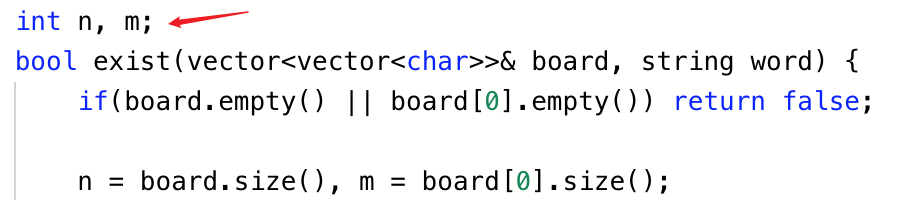2. 如何查看别人提交的代码
点开提交记录，不仅可以看到自己的历史代码，代码时空效率，还能看别人提交的效率很高的带点红色柱子就可以看别人的代码了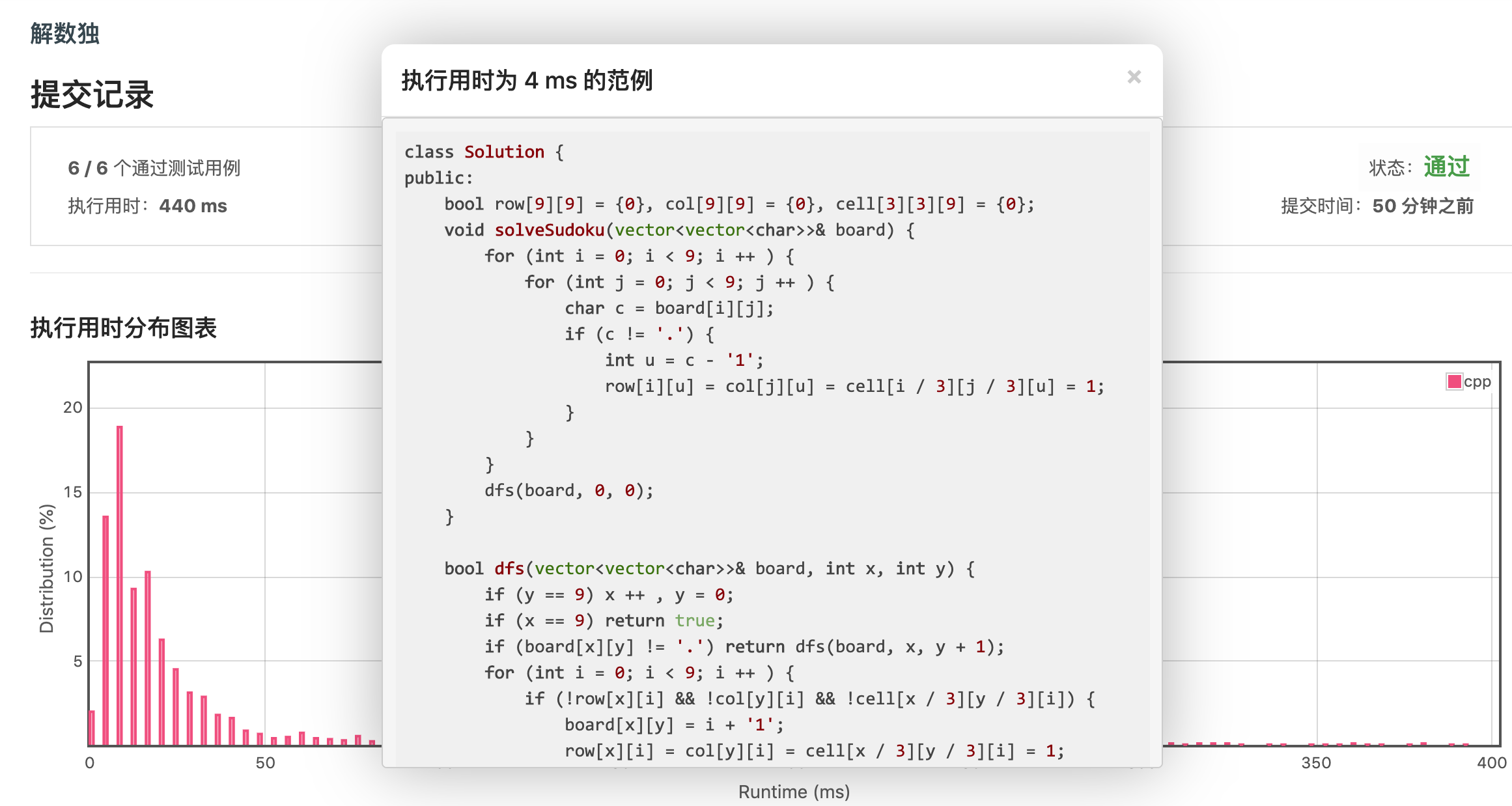3. LeetCode多组数据的坑点，新手必须要看！！！
最新万能解决方法：

全部放public内（包括#define,typedef,const)，只需注意const要加static
成员变量或函数间相对顺序任意，都是可以调用的
成员变量会自动初始化为0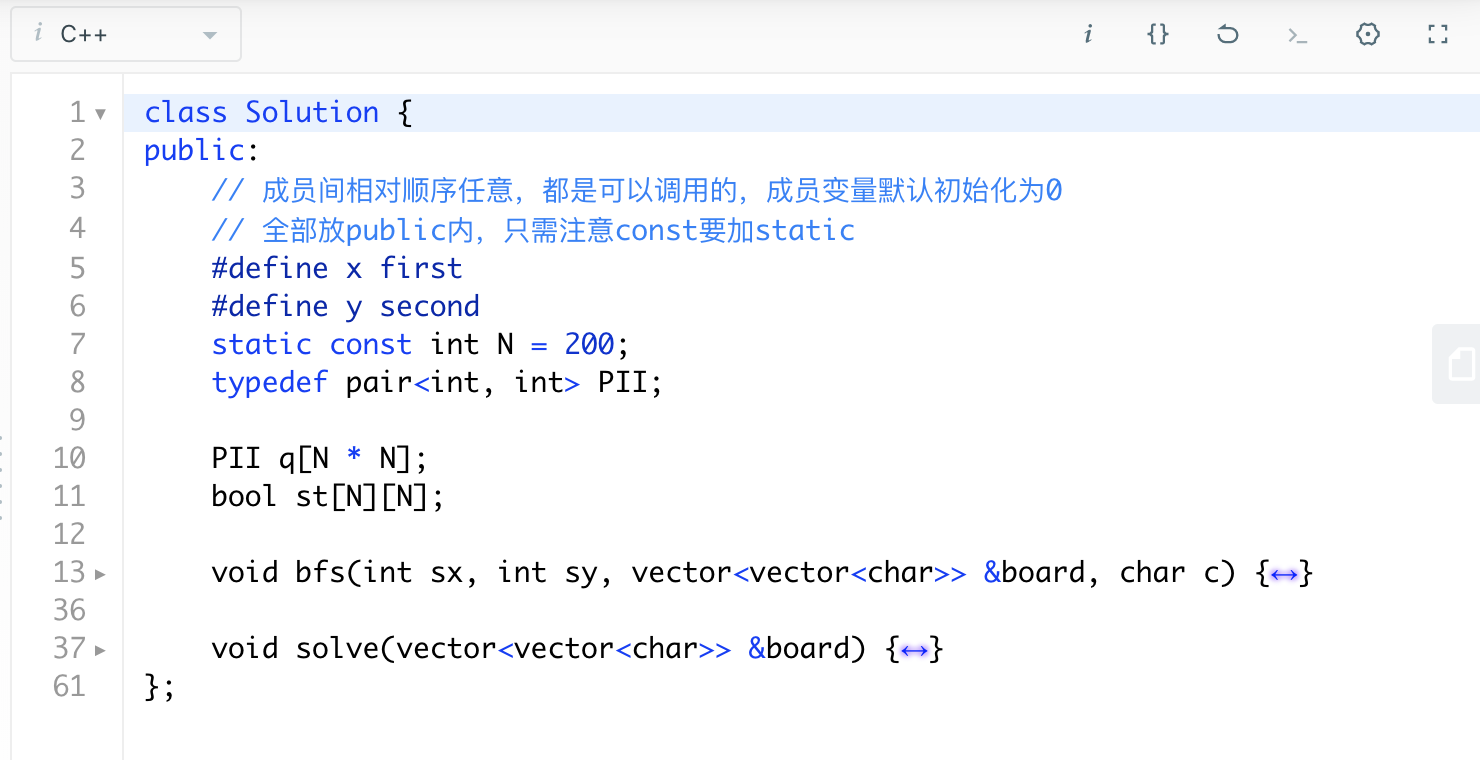力扣判题机，对每个测试用例都会初始化一次类

技术问题传送门
多组数据有问题，主要是因为力扣判题机，对每个测试用例都会初始化一次类，但全局变量，只会初始化第一次，后面需要手动初始化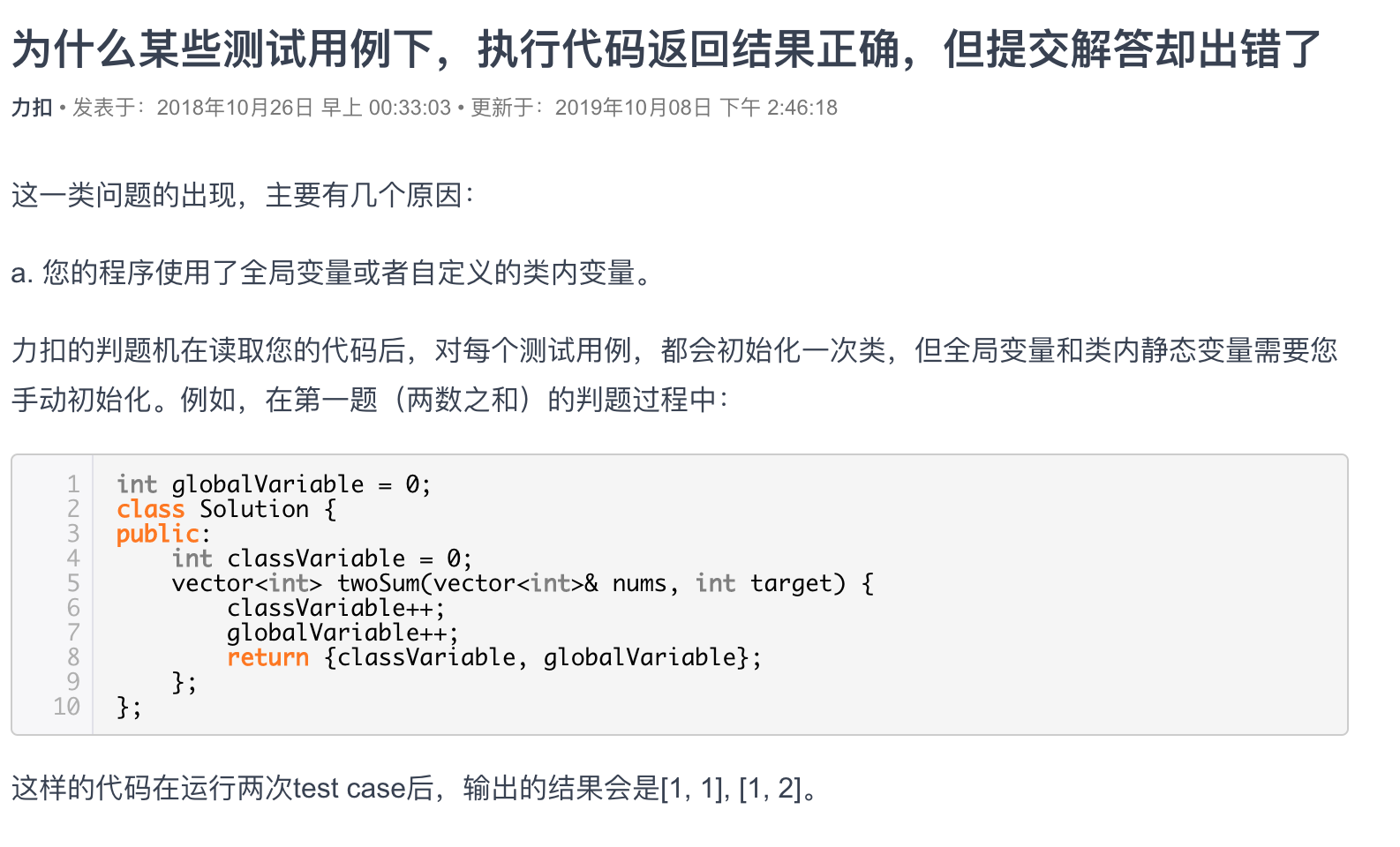4. vector等容器若不用clear清空，则必须放类内

当vector容器作为全局变量时因为LeetCode要测多组数据，所以必须要用clear清空vector
所以像vector，map这类容器最好定义为类内变量(因为每个测试样例都会初始化一次类)，否则你可能在本地测试是通过的，但在提交代码就不会通过，因为它测了多组数据，而vector，map等每次并没有清空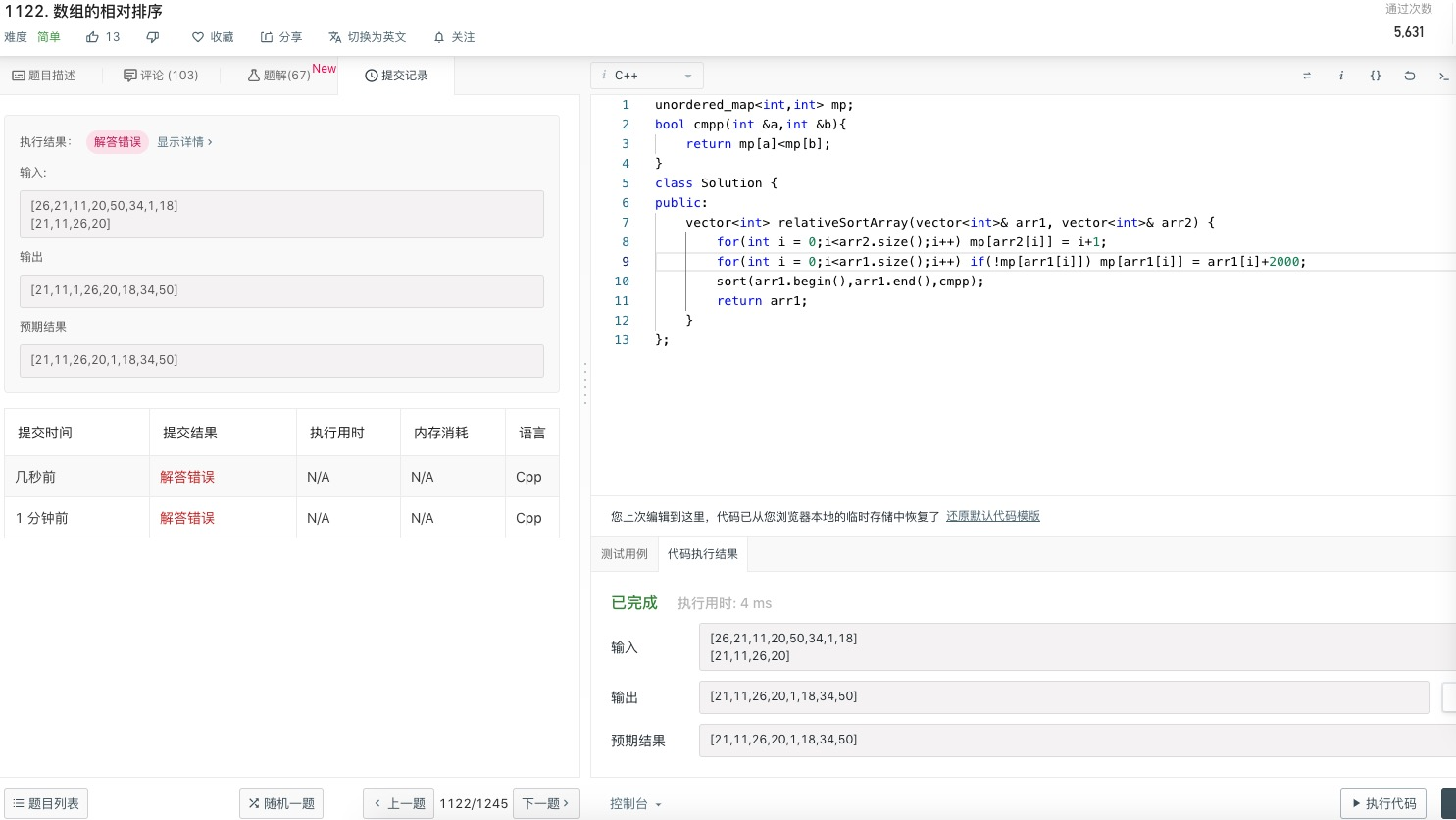5. 提交次数和用户尝试次数的含义
提交次数是指执行代码的次数，通过次数指执行代码成功的次数
用户尝试次数是指提交解答的次数，用户通过次数是指提交解答Accepted的次数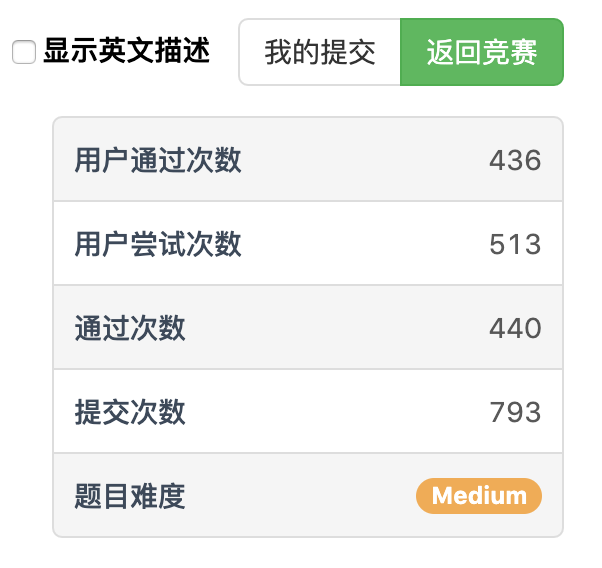6. 建议：在全局开vector数组+在函数内初始化
虽然习惯了在全局开一个常量数组，如int q[N]; ，但因为一开始可能不知道N要开多大，有可能开得太多，导致多一次提交错误
所以其实可以这样做（建议用vector）
情况1：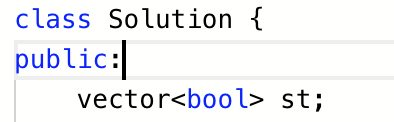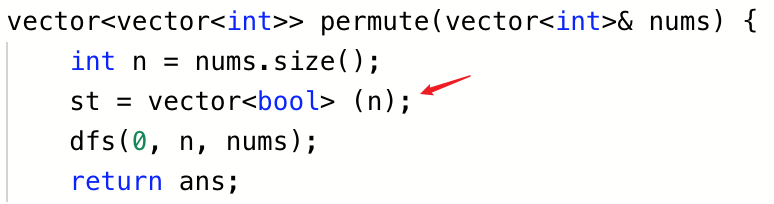情况2：
如果想把 $vector$设为“全局”的变量，方便你定义的其他函数调用时，您可以现在先在 $class$ 内定义，然后在题目给的函数中通过赋值的方法传给这个“全局”的变量
例如
class Solution {
public:
vector<vector<int>> f; // “全局”vector
int n, m;

// 题目给的函数
bool isMatch(string s, string p) {
n = s.size();
m = p.size();
// 通过赋值的方法给f传值
f = vector<vector<int>>(n + 1, vector<int>(m + 1, -1));
return dp(0, 0, s, p);
}

// 自定义函数
bool dp(int x, int y, string &s, string &p) {
// 这里f可以直接使用，不需要以参数的形式传入
if (f[x][y] != -1) return f[x][y];
...
}
};

示例代码：lc 329. 矩阵中的最长递增路径
class Solution {
public:
vector<vector<int>> f;
int dx = {0, -1, 0, 1}, dy = {1, 0, -1, 0};
int n, m;
// 记忆化搜索（dp的记忆化递归实现）
int dp(int x, int y, vector<vector<int>>& matrix) {
int &v = f[x][y];
if (v != -1) return v;

v = 1;
for (int d = 0; d < 4; d ++) {
int a = x + dx[d], b = y + dy[d];
if (0 <= a && a < n && 0 <= b && b < m && matrix[a][b] > matrix[x][y]) {
v = max(v, dp(a, b, matrix) + 1);
}
}
return v;
}

int longestIncreasingPath(vector<vector<int>>& matrix) {
n = matrix.size(), m = matrix.size();
f = vector<vector<int>> (n, vector<int>(m, -1));

int res = 0;
for (int i = 0; i < n; i ++) {
for (int j = 0; j < m; j ++) {
res = max(res, dp(i, j, matrix));
}
}
return res;
}
};

7. 错误提示pointer index
可能是输入的字符串没有加" "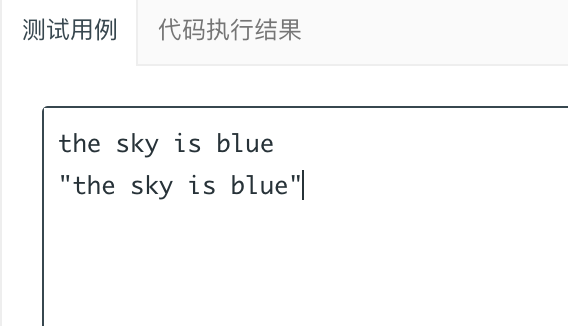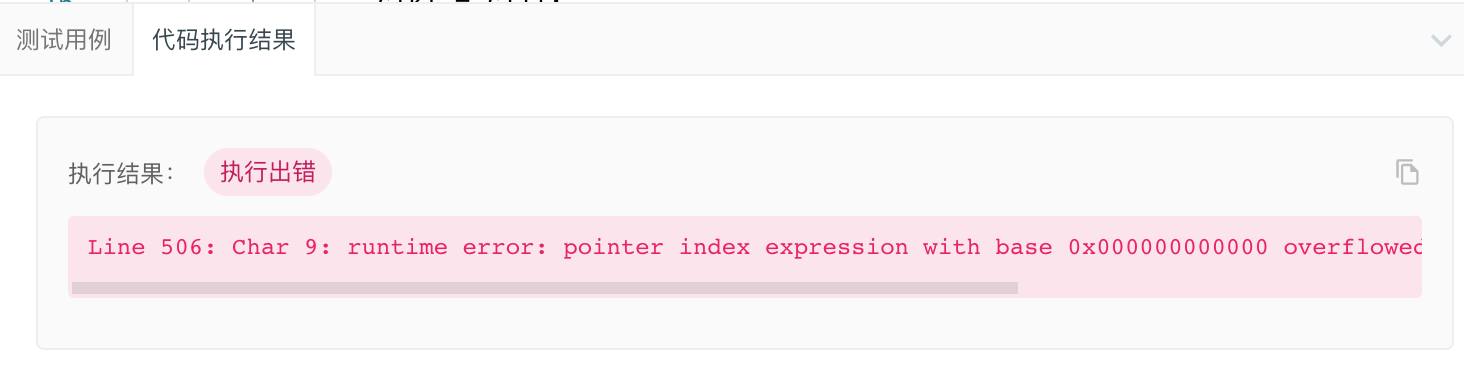内存使用超出范围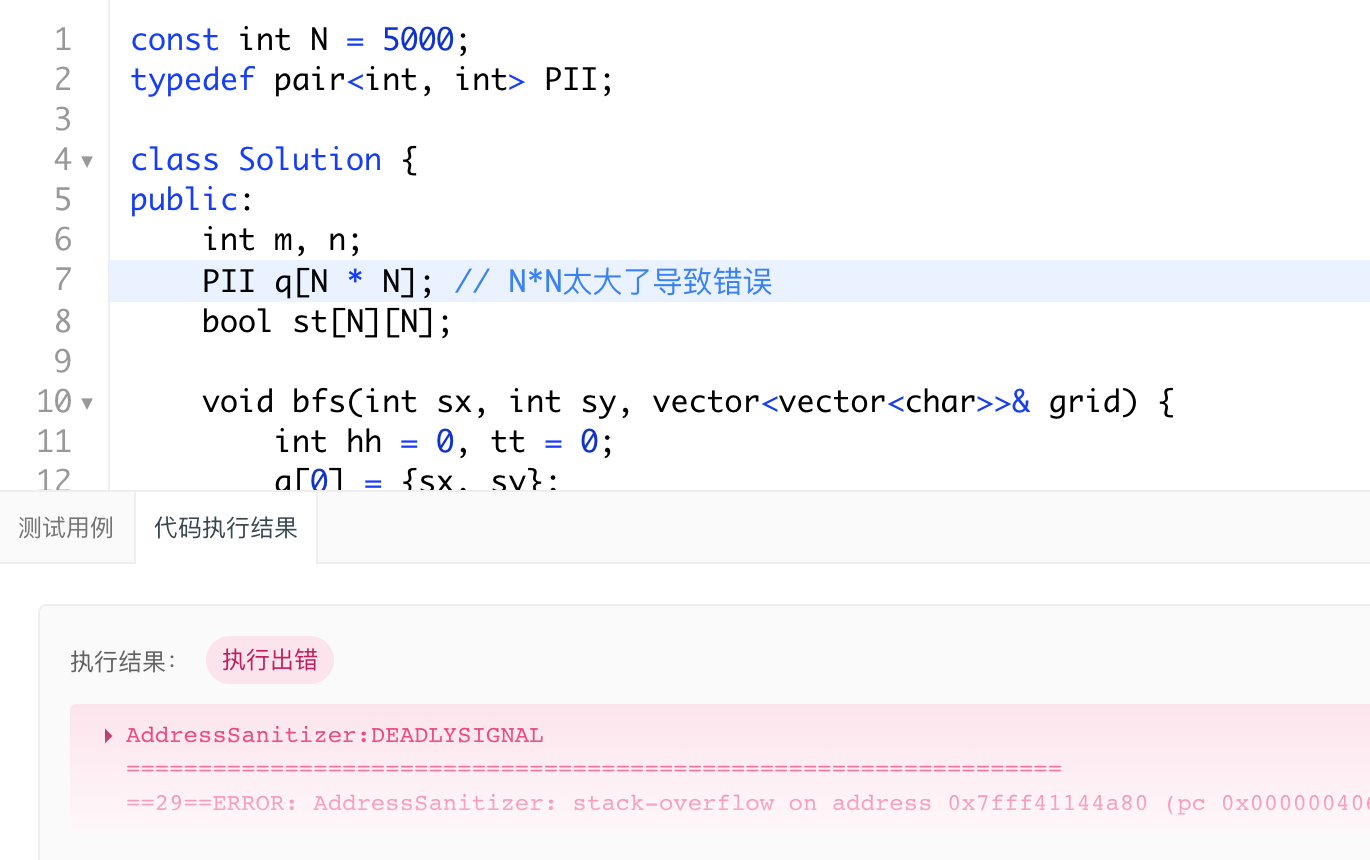9. C++ sort函数第三个参数cmp必须声明为static
你是否在刷LeetCode时出现过下面的情况？
而加上static就不会报错具体原因分析请看：https://ugirc.blog.csdn.net/article/details/104242037
10. const int M = 1e5报错：invalid use of non-static data member
1743. Restore the Array From Adjacent Pairs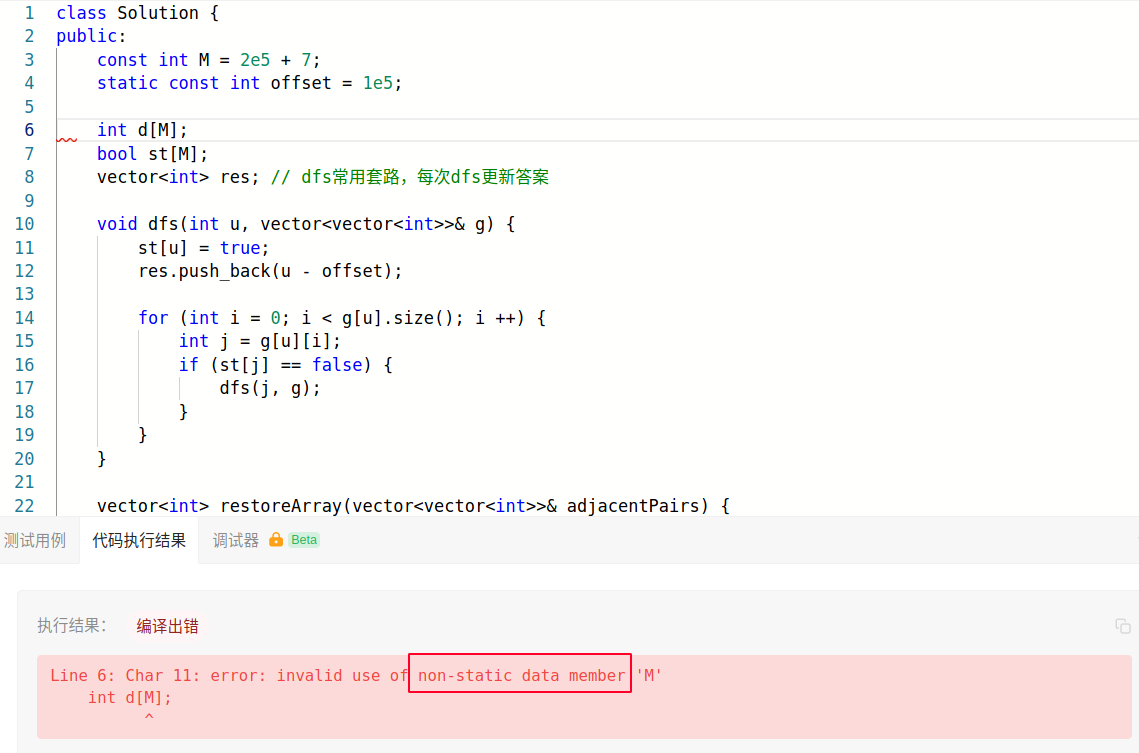LeetCode class Solution类内必须使用静态常量，即 static const int M = 2e5 + 7;
11. class类内vector val(N,0);初始化报错 C++ compile time error: expected identifier before numeric constant
vector作为public成员函数，如果要初始化大小，不能直接 vector val(N,0);
vector<int> val;                    // right

vector<int> val(N,0);               // error

vector<int> val{vector<int>(N,0)};  // right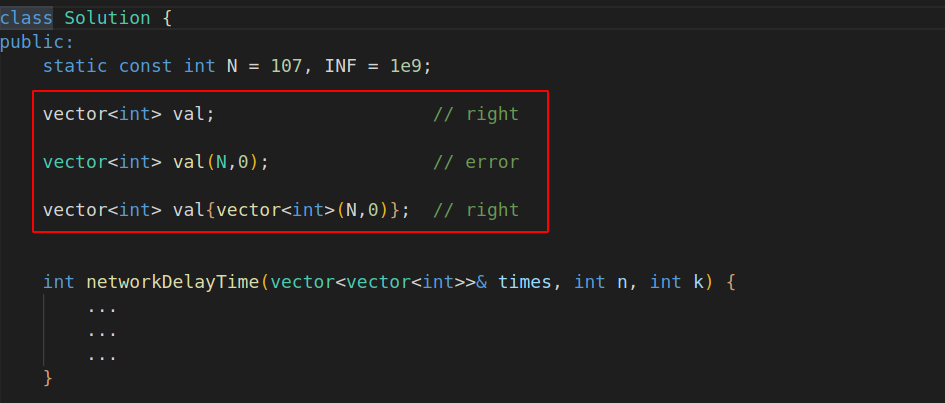参考：C++ compile time error: expected identifier before numeric constant
12. LeetCode只需你写函数，不用写完整的代码
在LeetCode你只需要写函数，头文件一般不用写，后台会帮你添加
完整代码可以在点playground查看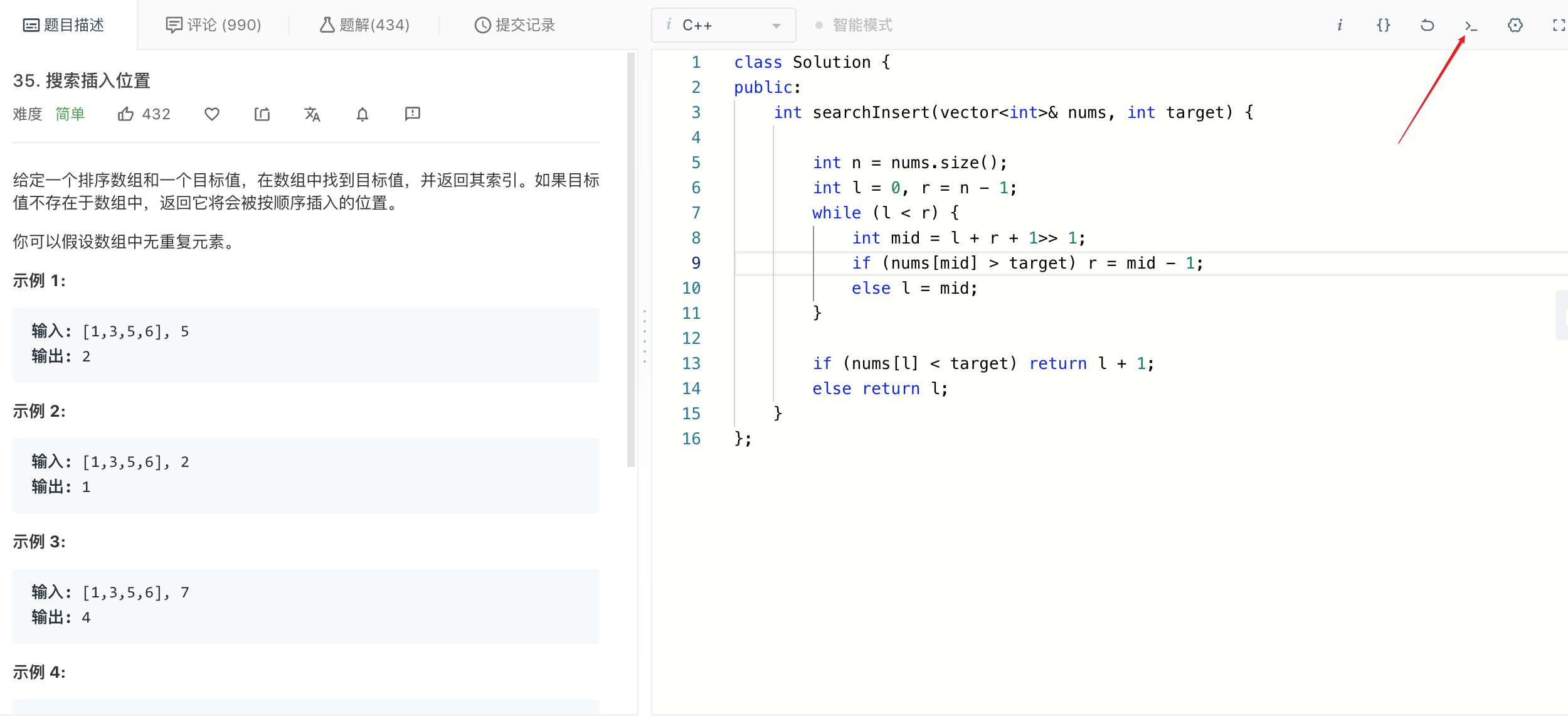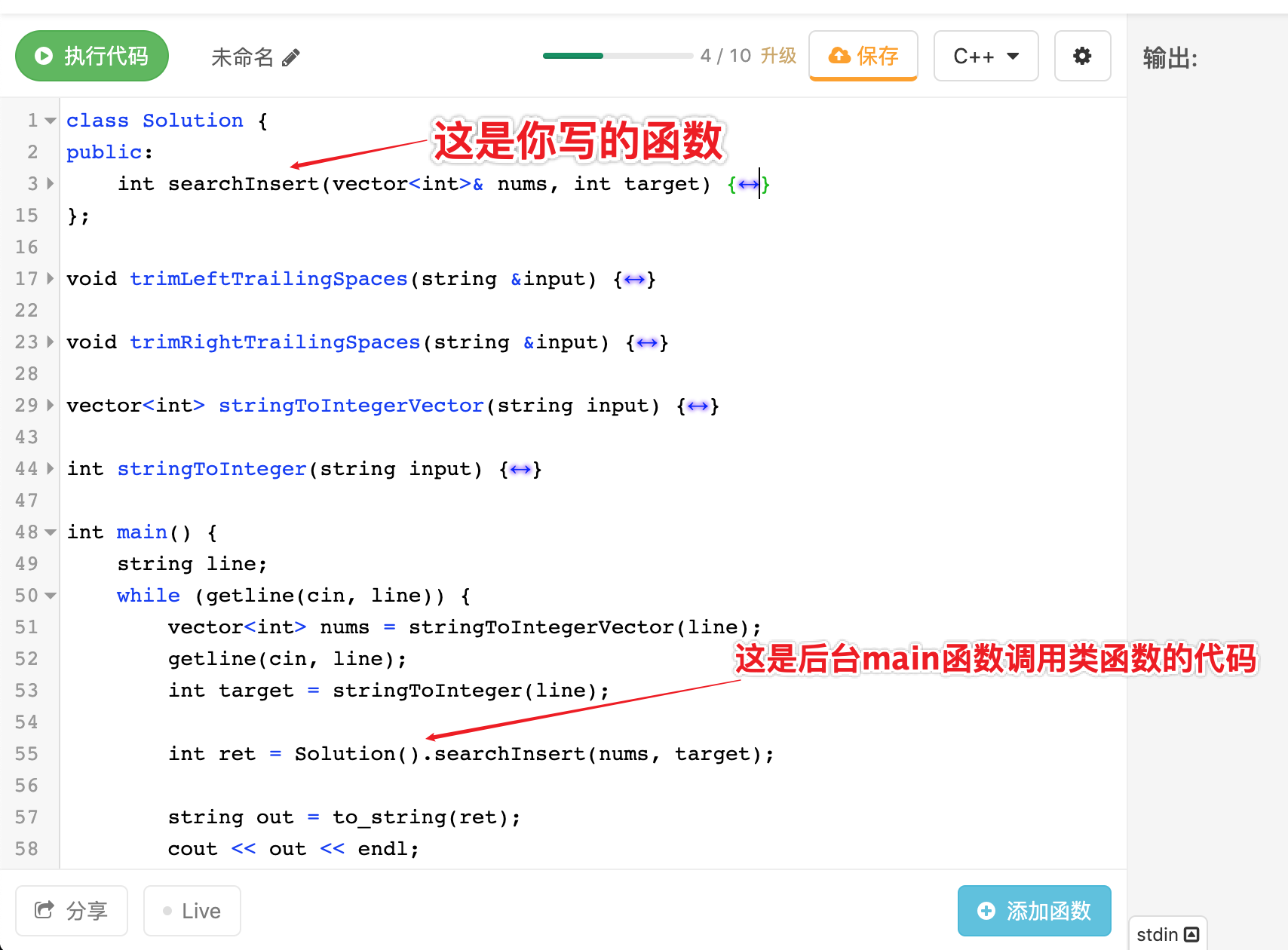代码示例：
class Solution {
public:
int searchInsert(vector<int>& nums, int target) {
int n = nums.size();
int l = 0, r = n - 1;
while (l < r) {
int mid = l + r + 1>> 1;
if (nums[mid] > target) r = mid - 1;
else l = mid;
}

if (nums[l] < target) return l + 1;
else return l;
}
};

void trimLeftTrailingSpaces(string &input) {
input.erase(input.begin(), find_if(input.begin(), input.end(), [](int ch) {
return !isspace(ch);
}));
}

void trimRightTrailingSpaces(string &input) {
input.erase(find_if(input.rbegin(), input.rend(), [](int ch) {
return !isspace(ch);
}).base(), input.end());
}

vector<int> stringToIntegerVector(string input) {
vector<int> output;
trimLeftTrailingSpaces(input);
trimRightTrailingSpaces(input);
input = input.substr(1, input.length() - 2);
stringstream ss;
ss.str(input);
string item;
char delim = ',';
while (getline(ss, item, delim)) {
output.push_back(stoi(item));
}
return output;
}

int stringToInteger(string input) {
return stoi(input);
}

int main() {
string line;
while (getline(cin, line)) {
vector<int> nums = stringToIntegerVector(line);
getline(cin, line);
int target = stringToInteger(line);

int ret = Solution().searchInsert(nums, target);

string out = to_string(ret);
cout << out << endl;
}
return 0;
}

相关文章：什么是核心代码模式，什么又是ACM模式？
13. LeetCode代码编辑器vim模式
LeetCode是支持vim模式的，虽然功能只有最基本的，也不支持vimrc，不过讲究着用吧，对于vim党来说有就不错了。
ctrl + c退出insert模式
esc会失去编辑器聚焦
另外windows会出现ctrl + c无法复制文本的问题，解决方法
14. LeetCode题目转为Markdown格式的实用插件安装 Chrome 插件 Leetcode Helper 后，只要点击  Copy for Markdown，它就会自动把题目的 Markdown 源码加到你剪贴板中把 Markdown 代码复制到你的博客中，这样你写博客时就方便了很多，不用再自己打一遍题目了15. 刷题必备 VScode/IDEA LeetCode插件配置（摸鱼神器）
如何在vscode当中配置leetcode插件？Clion / IDEA / Pycharm：leetcode-editor
LeetCode editor开箱体验——在 IntelliJ IDE中刷 Leetcode 题目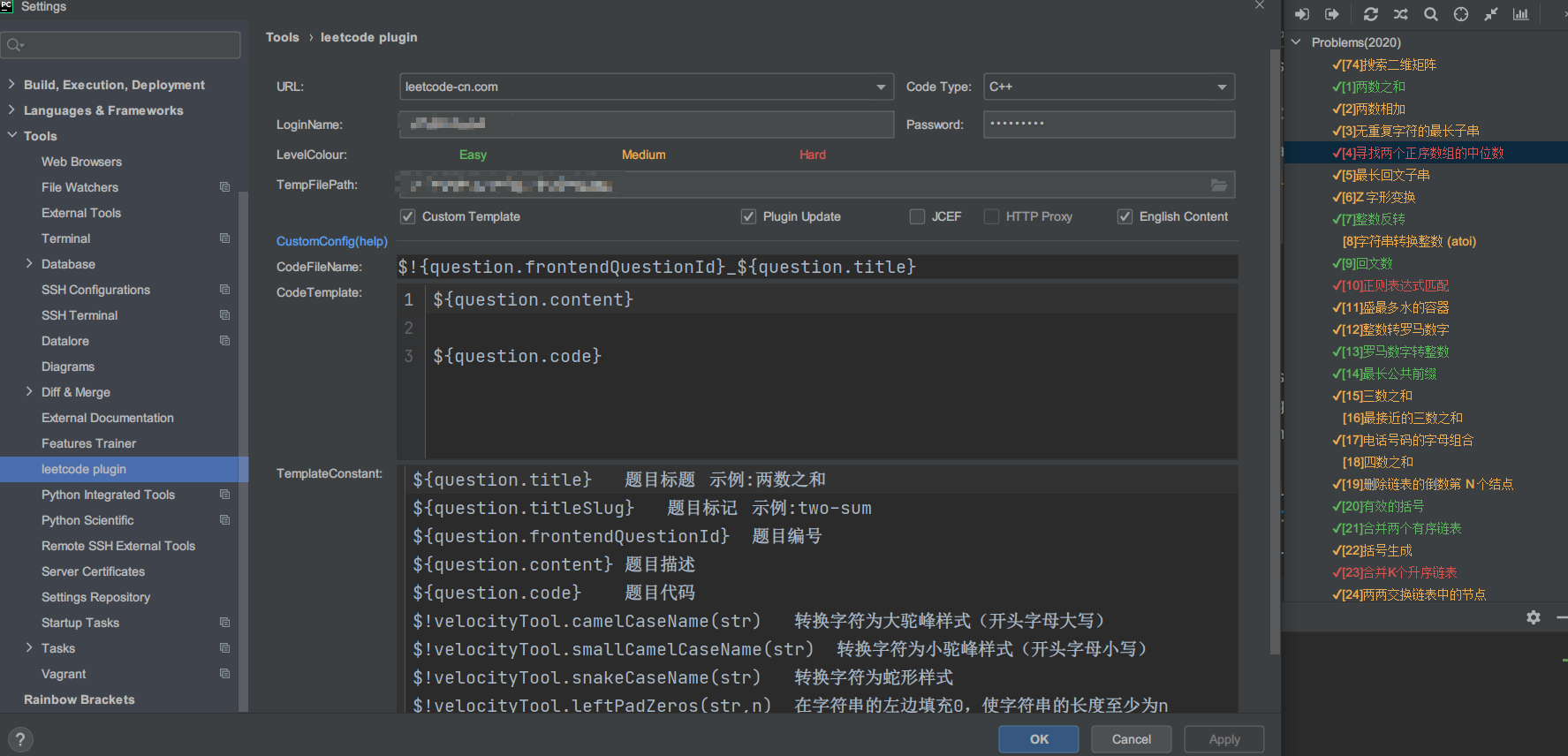16. 力扣个人页面的draw()函数如果想修改默认的字符，可以打开下面网站，直接生成自定义的字符
http://patorjk.com/software/taag
Related：
17. 力扣上的代码想在本地编译运行？
我一般会在本地用vim写LeetCode的题目，然后会写完整的程序，但是只是用来测试这个class Solution类你能不能顺利通过测试，如果想调试我还是会扔到LeetCode界面上用cout大法调试。主要是因为有些输入用例比较难写，比如二叉树，还要去构造棵树。
/**************************************************
> Author   : Anish Hui
> Blog     : https://ugirc.blog.csdn.net
> LastTime : 2021-03-31 Wed 10:37:40
**************************************************/
#include <iostream>
#include <vector>
using namespace std;

class Solution {
public:
bool searchMatrix(vector<vector<int>>& matrix, int target) {
int n = matrix.size(), m = matrix.size();
int l = 0, r = m - 1;
while (l < n && r >= 0) {
int x = matrix[l][r];
if (x > target) r --;
else if (x < target) l ++;
else return true;
}
return false;
}
};

int main() {

cout << "编译通过" << endl;

return 0;
}

相关文章：力扣上的代码想在本地编译运行？
附: Codeforces攻略
请参考下面的博客
Codeforces游玩攻略
Codeforces快速精通


展开全文• Leetcode 793. 阶乘函数后K个零 题目 f(x) 是 x! 末尾是0的数量。（回想一下 x! = 1 * 2 * 3 * … * x，且0! = 1） 例如， f(3) = 0 ，因为3! = 6的末尾没有0；而 f(11) = 2 ，因为11!= 39916800末端有2个0。给定 K...
• leetcode 分类 leetcode leetcode刷题记录，按照难度分类 每个题一个cpp文件，leetcode测试集通过 每个cpp文件的main函数实现对一个测试用例的测试，g++编译通过（c++11）
• leetcode 第28题 实现函数strStr 在主串中查找子串首次出现的位置我的 思路 是从主串第一个位置开始与子串匹配，如果当前字母不匹配，那么 主串 从 上次开始匹配的下一个位置 开始与子串的第一个字符开始匹配（这种...
• C++实现 leetcode做题，用于本地调试生成测试用例（即树）c++ 树结构
• ## leetcode

千次阅读 2019-09-04 19:50:58
leetcode题海游历一、leetcode题目1、整数反转 一、leetcode题目 leetcode题海游历。 1、整数反转 链接: link.
• 要用通用的测试工具，却又有杀鸡用牛刀的感觉，所以就写了个简单易用的leetcode开发测试工具，分享与大家。 工具安装 npm i leetcode_test 使用示例1 (问题010) codes: let test = require('leetcode_test').test /...
• 在刷leetcode题目的时候，我总结了一些编写测试用例的辅助函数，用于方便地生成和输出array, vector, list, tree, 希望对大家有帮助 二：API说明 1. 通用数据结构 //链表结点 struct ListNode { int val; ListNode...
• 项目使用 Bazel 构建的 C++ 刷题项目，通过几个简单步骤即可完成编译、测试，提供工具用于代码格式化、覆盖率报告、持续集成，还有额外的工具函数辅助创建测试用例。 如果你正使用 C++ 刷 LeetCode，但苦于每次写完...
• leetcode 答案 leetcode leetcode_practice 自己刷的leetcode，包含了自己想的实现和一些课程或者其他博客的参考答案。 也包括了乱七八糟注释和测试...按leetcode上的问题函数命名，或者有重名的可能是函数名+题号。...